Courses

# Test: Nuclear Chemistry - From Past 28 Years Questions

## 18 Questions MCQ Test Chemistry 28 Years Past year papers for NEET/AIPMT Class 12 | Test: Nuclear Chemistry - From Past 28 Years Questions

Description
This mock test of Test: Nuclear Chemistry - From Past 28 Years Questions for NEET helps you for every NEET entrance exam. This contains 18 Multiple Choice Questions for NEET Test: Nuclear Chemistry - From Past 28 Years Questions (mcq) to study with solutions a complete question bank. The solved questions answers in this Test: Nuclear Chemistry - From Past 28 Years Questions quiz give you a good mix of easy questions and tough questions. NEET students definitely take this Test: Nuclear Chemistry - From Past 28 Years Questions exercise for a better result in the exam. You can find other Test: Nuclear Chemistry - From Past 28 Years Questions extra questions, long questions & short questions for NEET on EduRev as well by searching above.
QUESTION: 1

Solution:
QUESTION: 2

### Emission of an alpha particle leads to a            

Solution:

Emission of α -particle )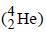leads to
decrease of 2 units of charge. e.g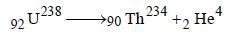QUESTION: 3

### If an isotope of hydrogen has two neutrons in itsatom, its atomic number and atomic mass numberwill respectively be    

Solution:

As number of neutron = Mass number
– atomic number
Give number of neutron = 2
∴ Mass number will be 3 and atomic number
will be one.

QUESTION: 4

In a radioactive decay, an emitted electron comesfrom 

Solution:

When a radioactive elements emits α or β
particle the new element formed may have
unstable nucleus. It may further disintegrate
by emitting α- or β particle forming a new
element. This process of integration may
continue till end product formed is a stable
compound.

QUESTION: 5

India has the world’s largest deposits of thoriumin the form of          

Solution:

The ore of thorium is monazite.

QUESTION: 6

Half-life for radioactive 14C is 5760 years. In howmany years, 200 mg of 14C will be reduced to 25mg?           

Solution:

Half-life of 14C = 5760 yrs; Initial weight of
14C = 200 mg and final weight of 14C = 25 mg.
Quantity left after 5760 years = 200/2  = 100 mg

Similarly quantity left after another 5760
years (i.e 11520 years) = 100/2 = 50 mg

Quantity left after another 5760 years
(i.e. 17280 years) = 50/2 = 25 mg

Thus time taken by 200 mg of 14C to reduce
to 25 mg = (5760 + 5760 + 5760 ) years = 17280
years.

Alternative solution

As we know that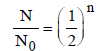where N0 original amount of radioactive
sustacnce
N = Amount of substance remain after n half
lives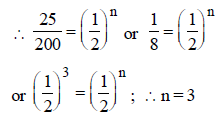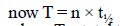where T = total time
T = 3 × 5760 years = 17280 years

QUESTION: 7

One microgram of radioactive sodium  2411Na witha half-life of 15 hours was injected into a livingsystem for a bio-assay. How long will it take forthe radioactive subtance to fall up to 25% of theinitial value? 

Solution: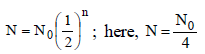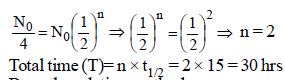QUESTION: 8

Carbon - 14 dating method is based on the factthat: 

Solution:

By carbon dating method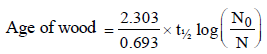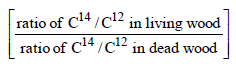Hence it is based upon the ratio of C14 and
C12.

QUESTION: 9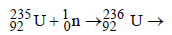fission products + neutrons + 3.20 × 10–11 J The energy released when 1 g of     finally undergoes fission is

Solution:

1 atom of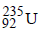on fission gives energy
= 3.2 × 10–11 J
6.023 × 1023 atom (1 mole) on fission gives
energy = 3.2 × 10–11 × 6.023 × 1023 J
235 gm ofon fission gives energy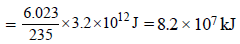QUESTION: 10

Number of neutrons in a parent nucleus X, which  gives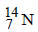nucleus, after two successive β emissions, would be

Solution: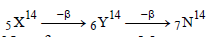No. of neutrons = Mass number – No. of
proton =14 – 5 = 9

QUESTION: 11

When a radioactive element emits successivelyone α-particle and two β-particles, the massnumber of the daughter element 

Solution:

Mass number is effected by emmision of α
particle while β particle has negligible mass
does not effect mass number. e.g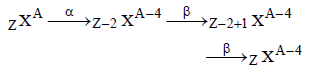QUESTION: 12

A human body required 0.01M activity ofradioactive substance after 24 hours. Half life ofradioactive substance is 6 hours. Then injectionof maximum activity of radioactive substance thatcan be injected will be 

Solution:

Remaining activity = 0.01M
after 24 hrs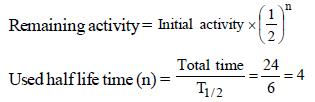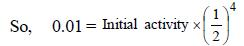Initial activity = 0.01 × 16 = 0.16M

QUESTION: 13

If species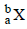emits firstly a positron, then two α and two β and in last one α and finally converted to species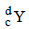, so correct relation is

Solution: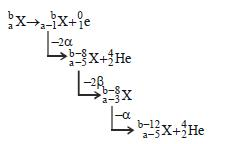QUESTION: 14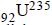nucleus absorbs a neutron and disintegrates into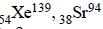and x. So what will be the product x? 

Solution: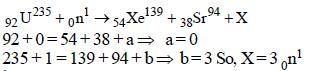i.e 3 neutrons

QUESTION: 15

The radioactive isotope, tritium,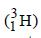has a halflifeof 12.3 years. If the initial amount of tritium is32 mg, how many milligrams of it would remainafter 49.2 years? 

Solution:

Given t1/2 = 12.3 years
Initial amount (N0) = 32 mg
Total time = 49.2 years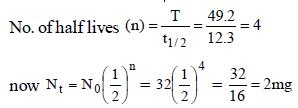Hence 32 mg becomes 2 mg in 49.2 years

QUESTION: 16

The radioactive isotope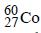which is used in the treatment of cancer can be made by (n, p) reaction. For this reaction the target nucleus is

Solution: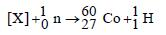Balancing the mass and atomic numbers on
both sides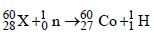Thus X should be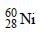QUESTION: 17

A nuclide of an alkaline earth metal undergoesradioactive decay by emission of the α-particles in succession. The group of theperiodic table to which the resulting daughterelement would belong is 

Solution:

When IIA group element (Ra) emits one
α-particle its group no. decreases by two
unit. i.e., go into zero group (Gr. 16) But as
it is radioactive thus due to successive
emission last product is Pb i.e., (Gr.14).

QUESTION: 18

The half life of a substance in a certain enzymecatalysedreaction is 138s. The time required forthe concentration of the substance to fall from1.28 mg L–1 to 0.04 mg L–1, is : 

Solution:

For a first order reaction
Total time T = no. of half lives (n) × half life
(t1/2)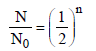where n = no. of half lives
Give N0 (original amount) = 1.28 mg/ ℓ
N (amount of substance left after time T)
= 0.04 m/g l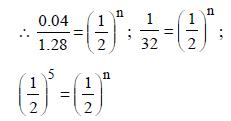n = 5
T = 5 × 138
= 690

Track your progress, build streaks, highlight & save important lessons and more!

### Similar Content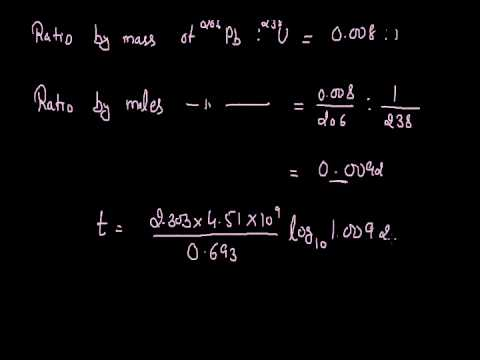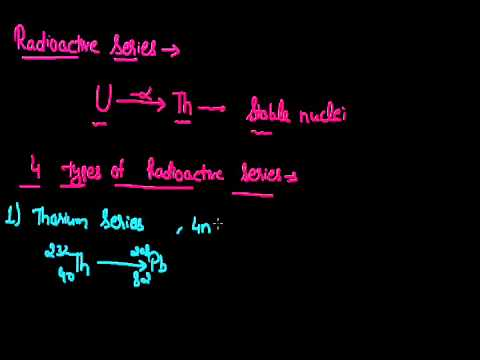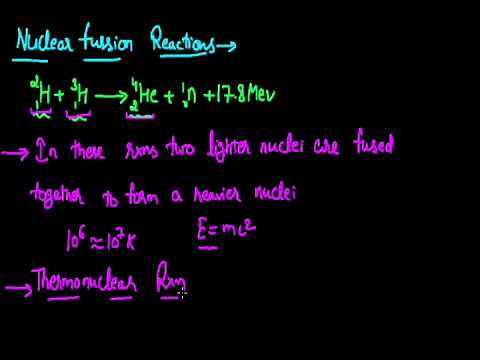### Related tests